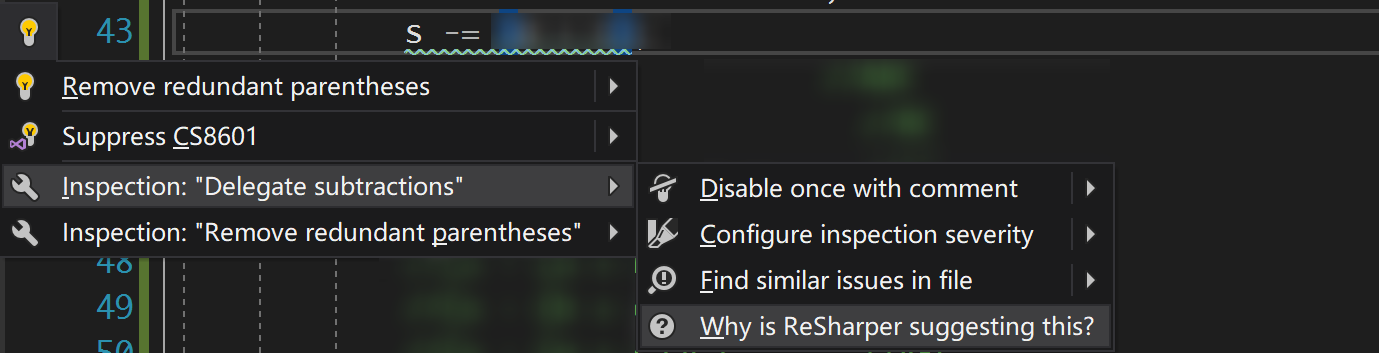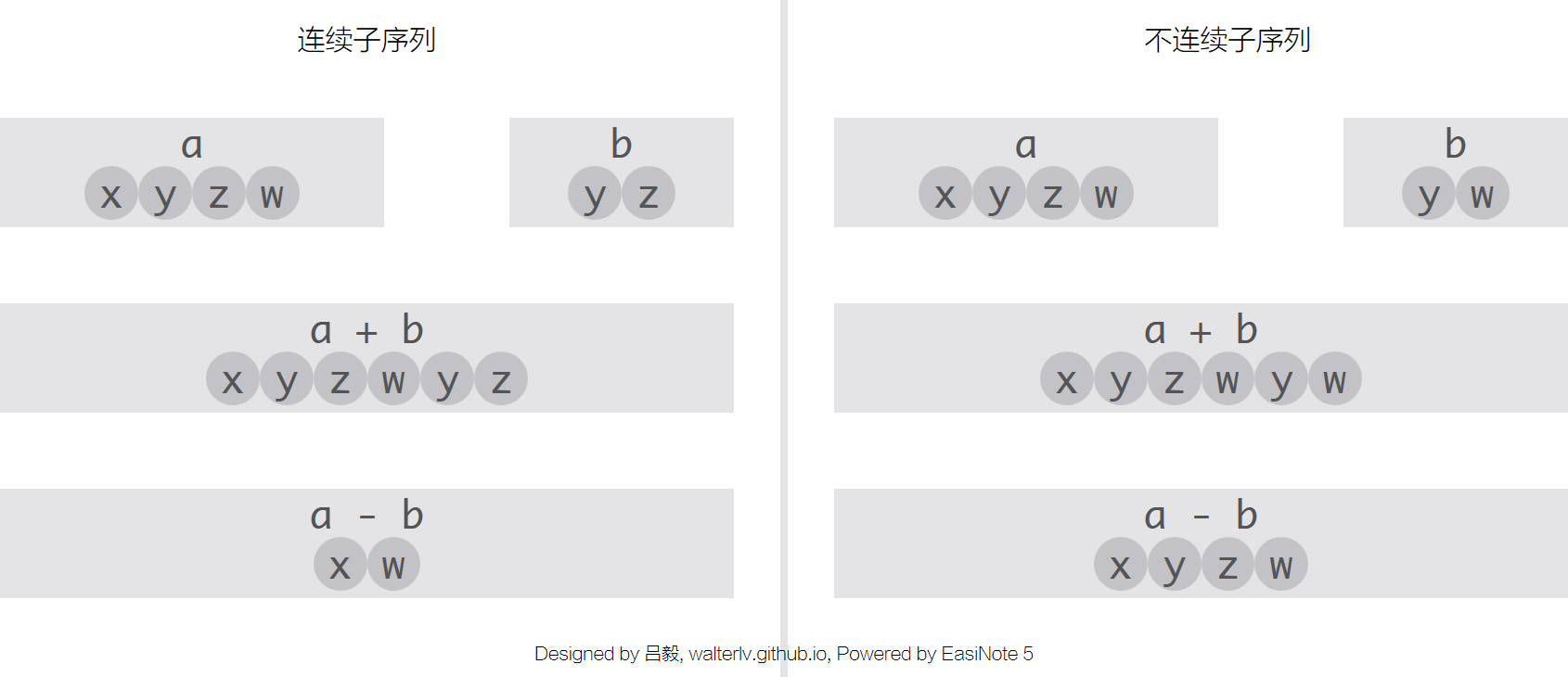# 为什么委托的减法（- 或 -=）可能出现非预期的结果？（Delegate Subtraction Has Unpredictable Result）▲ 委托的减法可能出现非预期的结果

## ReSharper 的官方帮助文档

### 例子和现象static void Main()
{
Action a = () => Console.Write("A");
Action b = () => Console.Write("B");
Action c = () => Console.Write("C");
Action s = a + b + c + Console.WriteLine;
s();                  //ABC
(s - a)();            //BC
(s - b)();            //AC
(s - c)();            //AB
(s - (a + b))();      //C
(s - (b + c))();      //A
(s - (a + c))();      //ABC
}


ReSharper 同时还给出另一个例子，说明委托的减法顺序也可能非预期：

s = a + b + a;
(s - a)();            // AB


### 原理▲ 调用列表的加减其实就是序列的拼接和子序列的移除

## 将委托和事件比较

private static event Action s;

static void Main()
{
Action a = () => Console.Write("A");
Action b = () => Console.Write("B");
Action c = () => Console.Write("C");
// 这一句注释掉，因为 s 换成了事件，而事件必须定义在类中。
// Action s = a + b + c + Console.WriteLine;
s();                  //ABC
(s - a)();            //BC
(s - b)();            //AC
(s - c)();            //AB
(s - (a + b))();      //C
(s - (b + c))();      //A
(s - (a + c))();      //ABC
}


## 不再用委托减法了吗？本作品采用 知识共享署名-非商业性使用-相同方式共享 4.0 国际许可协议 进行许可。欢迎转载、使用、重新发布，但务必保留文章署名 吕毅 （包含链接： https://blog.walterlv.com ），不得用于商业目的，基于本文修改后的作品务必以相同的许可发布。如有任何疑问，请 与我联系 ([email protected])